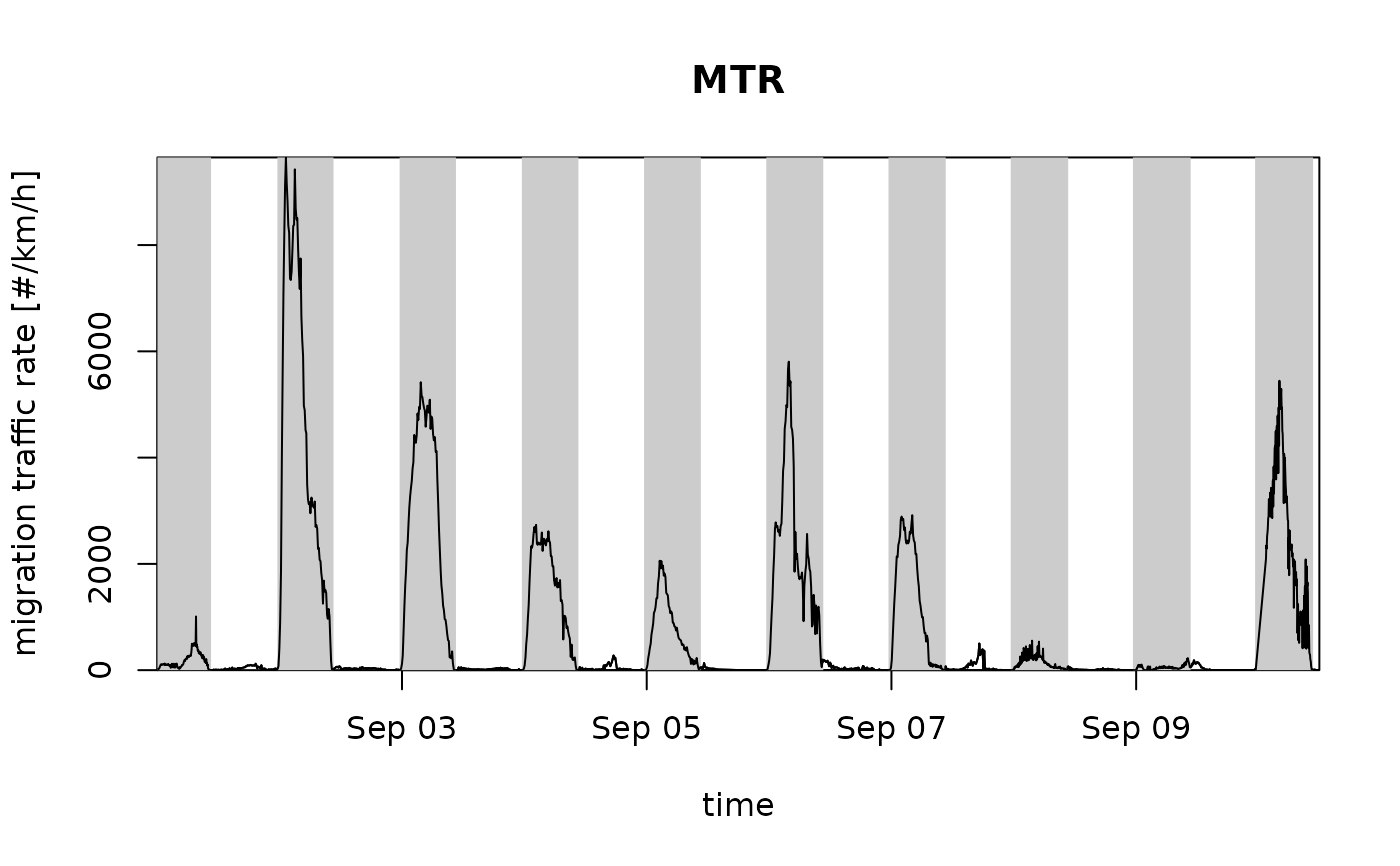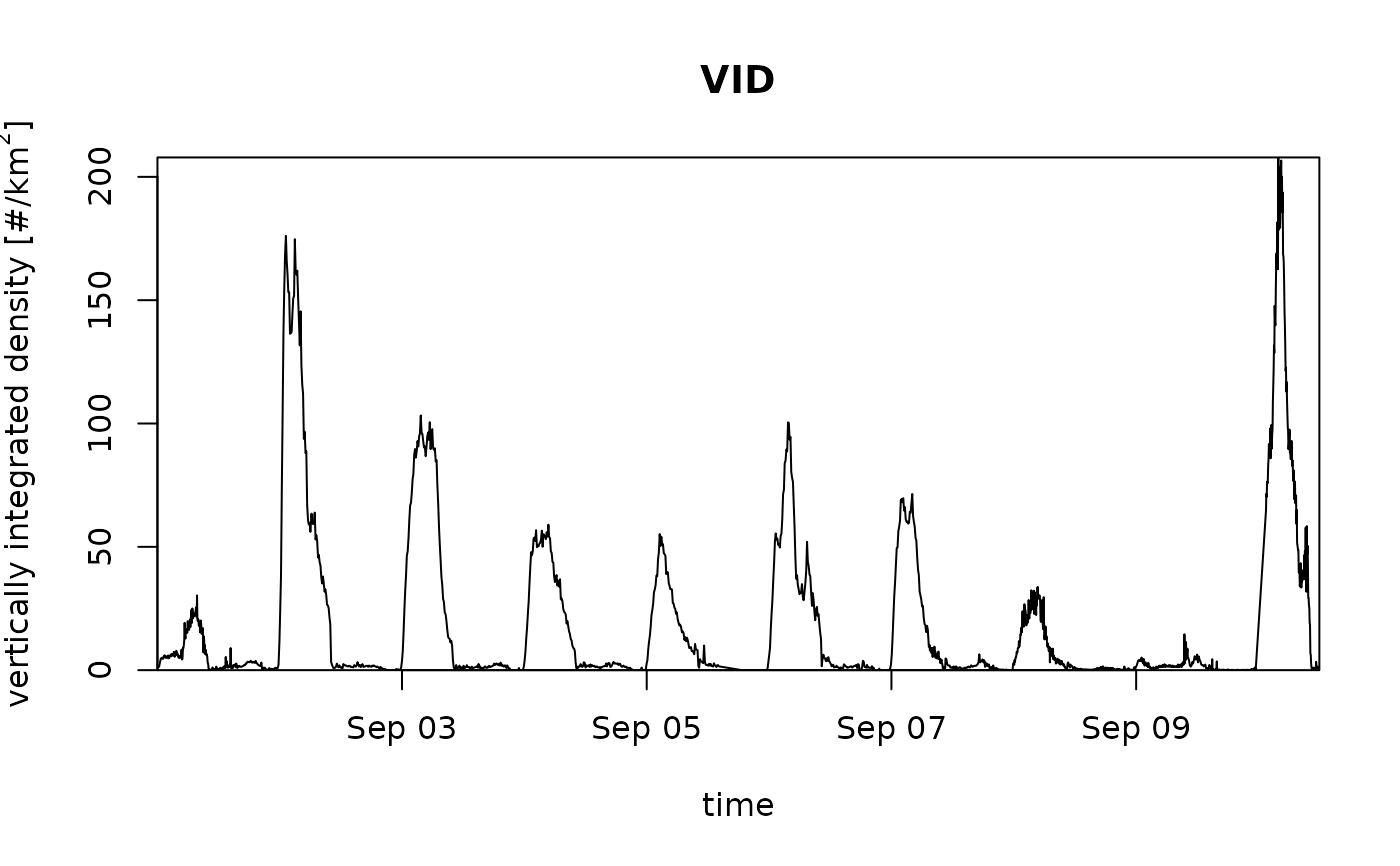Plot an object of class vpi.

# S3 method for vpi
plot(
x,
quantity = "mtr",
xlab = "time",
ylab = "migration traffic rate [#/km/h]",
main = "MTR",
elev = -0.268,
lat = NULL,
lon = NULL,
ylim = NULL,
...
)

## Arguments

x

1 class object inheriting from class vpi, typically a call to integrate_profile.

quantity

Character string with the quantity to plot, one of 'vid' (vertically integrated density), 'vir' (vertically integrated reflectivity), 'mtr' (migration traffic rate), 'rtr' (reflectivity traffic rate), 'mt' ((cumulative) migration traffic), 'rt' ((cumulative) reflectivity traffic), 'ff' (height-averaged ground speed) 'dd' (height-averaged direction) 'u' (height-averaged u-component of ground speed), 'v' (height-averaged v-component of ground speed).

xlab

A title for the x-axis.

ylab

A title for the y-axis.

main

A title for the plot.

Logical, whether to plot night time shading.

elev

Numeric, sun elevation to use for day/night transition, see sunrise.

lat

(optional) Latitude in decimal degrees. Overrides the lat attribute of x.

lon

(optional) Longitude in decimal degrees. Overrides the lon attribute of x.

ylim

y-axis plot range, numeric atomic vector of length 2.

...

Additional arguments to be passed to the low level plot plotting function.

## Details

The integrated profiles can be visualized in various related quantities, as specified by argument quantity:

"vid"

Vertically Integrated Density, i.e. the aerial surface density of individuals. This quantity is dependent on the assumed radar cross section per individual (RCS)

"vir"

Vertically Integrated Reflectivity. This quantity is independent of the value of individual's radar cross section

"mtr"

Migration Traffic Rate. This quantity is dependent on the assumed radar cross section (RCS)

"rtr"

Reflectivity Traffic Rate. This quantity is independent on the assumed radar cross section (RCS)

"mt"

Migration Traffic. This quantity is dependent on the assumed radar cross section (RCS)

"rt"

Reflectivity Traffic. This quantity is independent on the assumed radar cross section (RCS)

ff

Horizontal ground speed in m/s

dd

Horizontal ground speed direction in degrees

u

Ground speed component west to east in m/s

v

Ground speed component south to north in m/s

height

Mean flight height (height weighted by reflectivity eta) in m above sea level

The height-averaged ground speed quantities (ff,dd,u,v) and height are weighted averages by reflectivity eta.

## Examples

# vertically integrate a vpts object:
vpi <- integrate_profile(example_vpts)
# plot the migration traffic rates
plot(vpi)# plot the vertically integrated densities, without night shading:
plot(vpi, quantity = "vid", night_shade = FALSE)